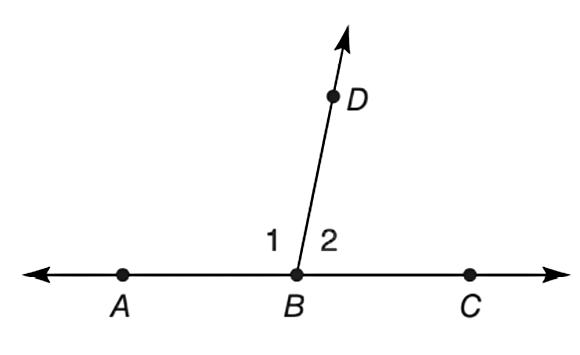Chapter 1.2, Problem 47E### Elementary Geometry for College St...

6th Edition
Daniel C. Alexander + 1 other
ISBN: 9781285195698

#### Solutions

Chapter
Section### Elementary Geometry for College St...

6th Edition
Daniel C. Alexander + 1 other
ISBN: 9781285195698
Textbook Problem
282 views

# In the figure, m ∠ 1 = x and m ∠ 2 = y . If x - y = 24 ° , find x and y .(HINT: m ∠ 1 + m ∠ 2 = 180 ° . )To determine

To find:

x and y for m1=x, m2=y and x-y=24°.

Explanation

Calculation:

Given,

m1=x,

m2=y and

x-y=24°(1)

In the above diagramABC is a straight angle, which is 180°.

Also the straight angle ABC is divided into two parts of angles by ABD and CBD.

Thus,

ABC=ABD+CBD

ABC=1+2

mABC=m=1+m2

180°=x+y

x+y=180°(2)

Now, add the equations (1) and (2) together

x-y+x+y=24°+180

### Still sussing out bartleby?

Check out a sample textbook solution.

See a sample solution

#### The Solution to Your Study Problems

Bartleby provides explanations to thousands of textbook problems written by our experts, many with advanced degrees!

Get Started

#### Evaluate the definite integrals in Problems 1-32. 24.

Mathematical Applications for the Management, Life, and Social Sciences

#### Differentiate. y=(z2+ez)z

Single Variable Calculus: Early Transcendentals, Volume I

#### In Exercises 4562, find the values of x that satisfy the inequality (inequalities). 48. 12 3x

Applied Calculus for the Managerial, Life, and Social Sciences: A Brief Approach

#### The harmonic series is: 1 + 2 + 3 + 4 + …

Study Guide for Stewart's Single Variable Calculus: Early Transcendentals, 8th